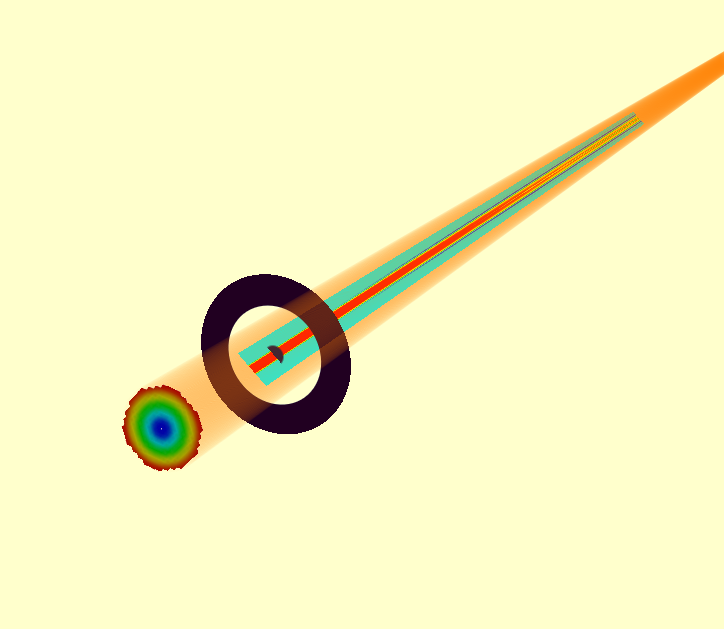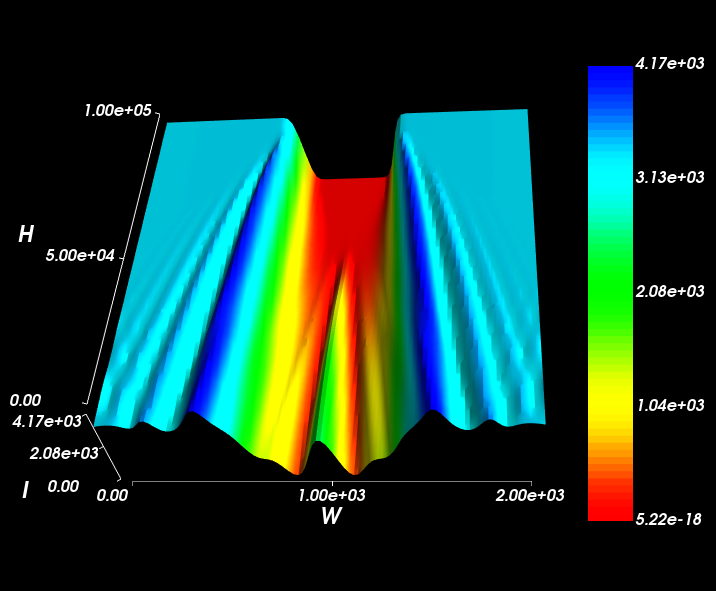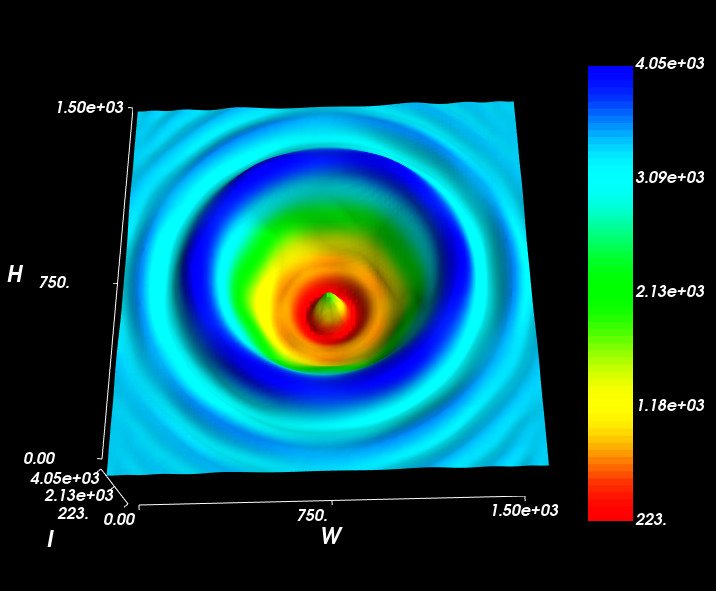Fresnel Diffraction From A BeamStop¶

We simply invert an aperture to create a beam-stop. Placing this the path of a collimated beam created interesting Fresnel-diffraction effects, including a bright spot at the centre of the blocked path, known as the Spot of Arago.

from raypier.tracer import RayTraceModel
from raypier.sources import HexagonalRayFieldSource, ConfocalRayFieldSource
from raypier.lenses import PlanoConvexLens
from raypier.apertures import CircularAperture
from raypier.fields import EFieldPlane
from raypier.constraints import BaseConstraint
from raypier.intensity_image import IntensityImageView
from raypier.intensity_surface import IntensitySurface

from traits.api import Range, on_trait_change
from traitsui.api import View, Item

aperture = CircularAperture(centre=(0,0,10), direction=(0,0,1),
hole_diameter = 0.5, edge_width=0.001, invert=True)

src = HexagonalRayFieldSource(resolution=10.0, direction=(0,0,1),
wavelength=1.0)

probe = EFieldPlane(source=src,
centre=(0,0,50),
direction=(0,1,0),
exit_pupil_offset=100.,
width=2.0,
height=100.0,
size=100)

img = IntensityImageView(field_probe=probe)
surf = IntensitySurface(field_probe=probe)

class FocalPlane(BaseConstraint):
z_pos = Range(50.0,130.0, 57.73)

traits_view = View(Item("z_pos"))

def __init__(self, *args, **kwds):
super().__init__(*args, **kwds)
self.on_change_z_pos()

@on_trait_change("z_pos")
def on_change_z_pos(self):
probe.centre = (0,0,self.z_pos)

model = RayTraceModel(sources=[src], optics=[aperture],
probes=[probe], constraints=[FocalPlane()],
results=[img, surf])

model.configure_traits()

The model looks as follows:However, the seem the detail in the Fresnel diffraction pattern, you need lots of Gausslets. Turn up the source resolution to at least 40.